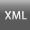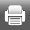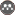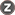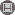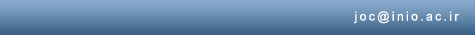[Home ] [Archive]   [ فارسی ]Volume 1, Issue 3 (2010)joc 2010, 1(3): 35-44 Back to browse issues page
A two-dimensional vertical non-hydrostatic model for simulation of free surface flows
Fatemeh Chegini , Masoud Montazeri Namin
, cheginif@ut.ac.ir
Abstract:   (12885 Views)
In this paper, details of the development of a two-dimensional vertical numerical model for simulating unsteady free-surface flows, deploying a non-hydrostatic pressure distribution is given. The governing 2DV Navier-Stokes equations are discretized using the finite volume approximation in the Cartesian coordinate and solved based on the algorithm of the projection method. Moreover, the model calculates eddy viscosity and diffusivity using the turbulence equations. In order to predict the moving free surface elevations, the height of the top layer is assumed to be variable and proportional to the water elevation. One of the advantages of the developed model is its higher computational speed compared to similar models developed in sigma and curvilinear coordinates. Therefore, in conditions that the variation of water and bed level is not considerable, this model is more appropriate. The model has been applied to simulate a range of unsteady flow problems involving relatively strong vertical accelerations. Comparison between numerical results, analytical solutions and experimental data demonstrates a satisfactory performance.
Keywords: LDV numerical model, Emplicit finite volume method, Free surface flow, Nonhydrostatic pressure
Type of Study: Research |
Received: 2012/01/13 | Accepted: 2016/10/18 | ePublished: 2016/10/18
Send email to the article author